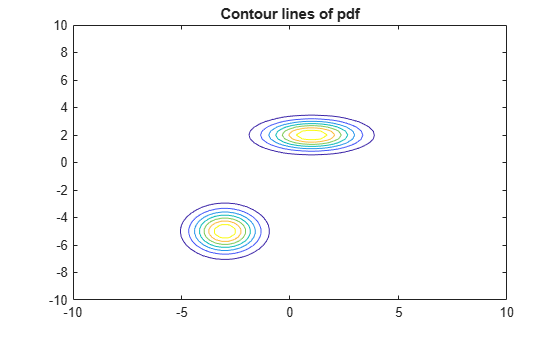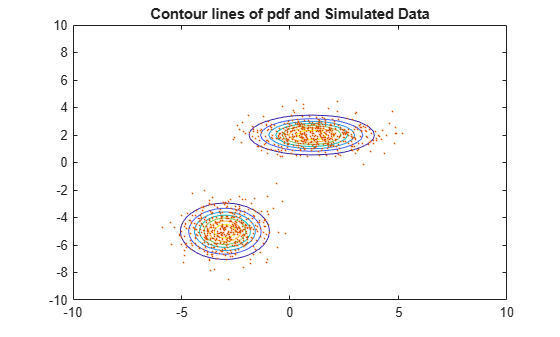# Simulate Data from Gaussian Mixture Model

This example shows how to simulate data from a Gaussian mixture model (GMM) using a fully specified `gmdistribution` object and the `random` function.

Create a known, two-component GMM object.

```mu = [1 2;-3 -5]; sigma = cat(3,[2 0;0 .5],[1 0;0 1]); p = ones(1,2)/2; gm = gmdistribution(mu,sigma,p);```

Plot the contour of the pdf of the GMM.

```gmPDF = @(x,y) arrayfun(@(x0,y0) pdf(gm,[x0 y0]),x,y); fcontour(gmPDF,[-10 10]); title('Contour lines of pdf');```Generate 1000 random variates from the GMM.

```rng('default') % For reproducibility X = random(gm,1000);```

Plot the variates with the pdf contours.

```hold on scatter(X(:,1),X(:,2),10,'.') % Scatter plot with points of size 10 title('Contour lines of pdf and Simulated Data')```